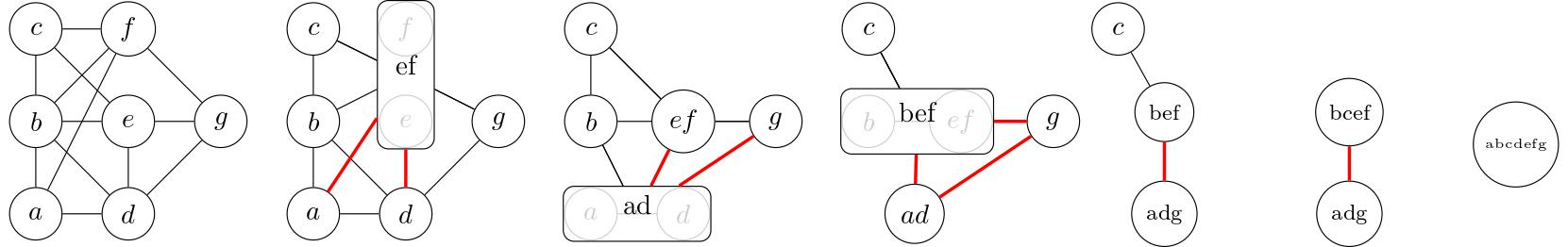TWIN-WIDTH, ANR JCJC project (21-CE48-0014-01)
A trigraph is a vertex set together with two disjoint edge sets: the black edges and the red edges. If a black edge can be thought of as a full adjacency between its endpoints, and a non-edge, as a full non-adjacency, a red edge is a mixed state between the former two. Two vertices are called black neighbors (resp. red neighbors) if they are linked by a black edge (resp. a red edge). In a trigraph, a contraction of two (non-necessarily adjacent) vertices, say, u and v, merges the two vertices into a single vertex, say, w and link it with a black edge to vertices that are black neighbors of both u and v, and with a red edge to all the other neighbors of u or v.A contraction sequence of an n-vertex (tri)graph G is a list of trigraphs G=Gn, Gn-1, ..., G2, G1 such that Gi is obtained from Gi+1 by performing one contraction. In particular G1 is the unique simple 1-vertex graph. A d-sequence is a contraction sequence such that the maximum red degree (that is, considering only the red edges) of every trigraph of the sequence is at most d. Finally the twin-width of a graph is the minimum integer d such that it admits a d-sequence. The following picture represents a contraction sequence witnessing that the initial 7-vertex (tri)graph has twin-width at most 2.Classes with bounded twin-width: bounded cliquewidth classes, proper-minor closed classes, unit interval graphs, proper hereditary permutation classes, every graph whose edges are subdivided at least logarithmically many times, iterated O(1)-lifts, biclique-free segment graphs

Classes with unbounded twin-width: subcubic graphs, interval graphs, segment graphs, subdivisions of n-cliques where each edge is subdivided between one and o(log n) times, visibility graphs of simple polygons or even of 1.5D terrains

What is twin-width good for?

Contraction sequences are algorithmically useful: linear FPT algorithm for checking a first-order sentence on a graph (parameterized by the twin-width bound and the size of the first-order sentence), improved approximation algorithms for Minimum Dominating Set, Maximum Independent Set and Coloring

Structural properties of bounded twin-width classes: 𝛘-boundedness, even quasipolynomial 𝛘-boundedness, smallness (i.e., only exponentially many graphs on a given slice; extends, while using it, the Marcus-Tardos theorem)

Twin-width and finite model theory: Bounded twin-width is preserved by operating first-order transductions (useful fact to show that classes have or have not bounded twin-width), in some classes (see delineation in the Open Questions), like ordered graphs and tournaments, bounded/unbounded twin-width coincides with the tractability limit for first-order model checking.

The lens of contraction sequences: Adding additional (or different) constraints to the red graphs of the sequence, one retrieves the classical cliquewidth and linear cliquewidth, as well as a handful of new parameters interpolating between cliquewidth and twin-width, and one may recast Courcelle's theorems in an angle of view that unifies MSO and FO model checking algorithms.